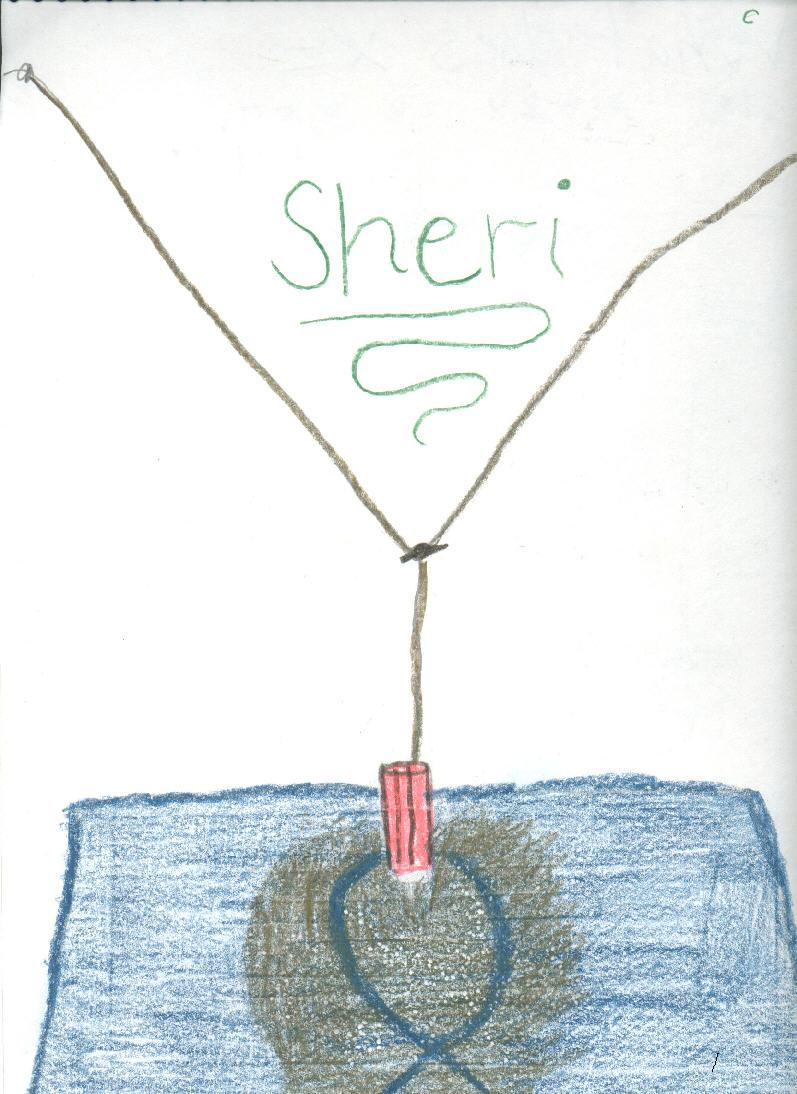Sheri draws the complex sand pendulum pattern

Don set up the complex sand pendulum in the math room. The strings at the top are adjustable so one can change the total height of the pendulum (pendulum #1). The strings come together to form a smaller pendulum (pendulum #2) and are held together by an alligator clip so this height can be changed. The sand is in a ketchup bottle. When the ketchup bottle is pulled off center, the pendulums will swing, perpendicularly, forming "Lissajous" figures like the one shown in Sheri's drawing. The pattern in the sand is determined by the ratio of the frequencies of the 2 pendulums (in this case 3:2).Sheri writes 64 using exponents
Sheri solves the quadratic equation x2 - x - 1 = 0
Sheri finds the base for Don's age of   114? = 7110
Sheri uses the quadratic formula to find base for Don's age of   114? = 7110
Sheri finds the measure of an inscribed angle
Sheri uses binary numerals to make the Magic Number Game cards
Sheri changes the shape of a dog using matrices
Sheri reduces the size of a shell using the pantograph
Sheri moves a parabola and finds the equation
Sheri finds the ratio of The Volume of a Pyramid / The Volume of a Cube (3 ways)
Sheri figures out a rule for The Tower Puzzle
Sheri starts Trig

To other discoveries

To order Don's materials
Mathman home